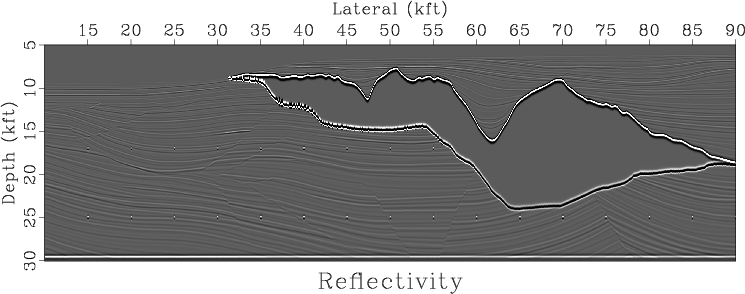sfai2refl converts acoustic impedance to normal-incidence PP reflectivity using the simple equation
$$R(t) = \frac{I(t+\Delta t) – I(t)}{I(t+\Delta t) + I(t)}$$

The program is useful for convolution modeling. The following example from rsf/rsf/wedge shows a classic example of wedge modeling, which is used for analyzing the tuning phenomenon (Kallweit, R. and L. Wood, 1982, The limits of resolution of zero-phase wavelets: Geophysics, v. 47, 1035-1046.)A more sophisticated example from geo384w/hw5/sigsbee2 computes an effective reflectivity of the Sigsbee model:The program has no parameters.